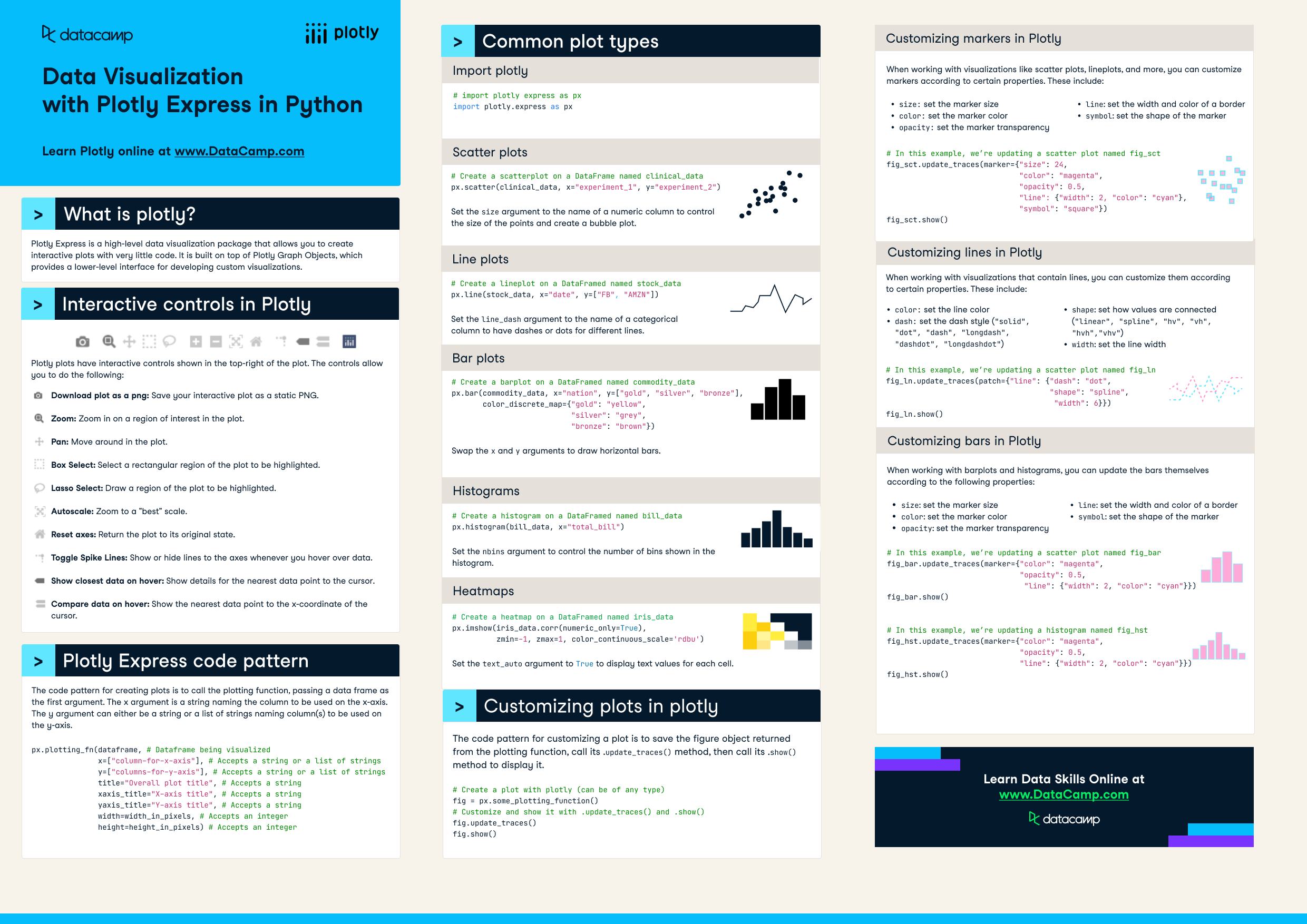# Plotly Express Cheat Sheet

Plotly is one of the most widely used data visualization packages in Python. Learn more about it in this cheat sheet.
Nov 2022  · 0 min read

## What is plotly?

Plotly express is a high-level data visualization package that allows you to create interactive plots with very little code. It is built on top of Plotly Graph Objects, which provides a lower-level interface for developing custom visualizations. This cheat sheet covers all you need to know to get started with plotly in Python.Have this cheat sheet at your fingertips

## Interactive controls in Plotly

Plotly plots have interactive controls shown in the top-right of the plot. The controls allow you to do the following:

• Pan: Move around in the plot.
• Box Select: Select a rectangular region of the plot to be highlighted.
• Lasso Select: Draw a region of the plot to be highlighted.
• Autoscale: Zoom to a "best" scale.
• Reset axes: Return the plot to its original state.
• Toggle Spike Lines: Show or hide lines to the axes whenever you hover over data.
• Show closest data on hover: Show details for the nearest data point to the cursor.
• Compare data on hover: Show the nearest data point to the x-coordinate of the cursor.

## Plotly Express code pattern

The code pattern for creating plots is to call the plotting function, passing a data frame as the first argument. The x argument is a string naming the column to be used on the x-axis. The y argument can either be a string or a list of strings naming column(s) to be used on the y-axis.

``````px.plotting_fn(dataframe, # Dataframe being visualized
x=["column-for-x-axis"], # Accepts a string or a list of strings
y=["columns-for-y-axis"], # Accepts a string or a list of strings
title="Overall plot title", # Accepts a string
xaxis_title="X-axis title", # Accepts a string
yaxis_title="Y-axis title", # Accepts a string
width=width_in_pixels, # Accepts an integer
height=height_in_pixels) # Accepts an integer``````

## Common plot types

``````# First import plotly express as px
import plotly.express as px``````

### Scatter plots

``````# Create a scatterplot on a DataFrame named clinical_data
px.scatter(clinical_data, x="experiment_1", y="experiment_2")``````

Set the `size` argument to the name of a numeric column to control the size of the points and create a bubble plot.

### Line plots

``````# Create a lineplot on a DataFramed named stock_data
px.line(stock_data, x="date", y=["FB", "AMZN"])``````

Set the `line_dash` argument to the name of a categorical column to have dashes or dots for different lines.

### Bar plots

``````# Create a barplot on a DataFramed named commodity_data
px.bar(commodity_data, x="nation", y=["gold", "silver", "bronze"],
color_discrete_map={"gold": "yellow",
"silver": "grey",
"bronze": "brown"})``````

Swap the `x` and `y` arguments to draw horizontal bars.

### Histograms

``````# Create a histogram on a DataFramed named bill_data
px.histogram(bill_data, x="total_bill")``````

Set the `nbins` argument to control the number of bins shown in the histogram.

### Heatmaps

``````# Create a heatmap on a DataFramed named iris_data
px.imshow(iris_data.corr(numeric_only=True),
zmin=-1, zmax=1, color_continuous_scale='rdbu')``````

Set the `text_auto` argument to `True` to display text values for each cell.

## Customizing plots in plotly

The code pattern for customizing a plot is to save the figure object returned from the plotting function, call its `.update_traces()` method, then call its `.show()` method to display it.

``````# Create a plot with plotly (can be of any type)
fig = px.some_plotting_function()

# Customize and show it with .update_traces() and .show()
fig.update_traces()
fig.show()
``````

### Customizing markers in Plotly

When working with visualizations like scatter plots, lineplots, and more, you can customize markers according to certain properties. These include:

• `size`: set the marker size
• `color`: set the marker color
• `opacity`: set the marker transparency
• `line`: set the width and color of a border
• `symbol`: set the shape of the marker
``````# In this example, we’re updating a scatter plot named fig_sct
fig_sct.update_traces(marker={ "size" : 24,
"color": "magenta",
"opacity": 0.5,
"line": {"width": 2, "color": "cyan"},
"symbol": "square"})
fig_sct.show()``````

### Customizing lines in Plotly

When working with visualizations that contain lines, you can customize them according to certain properties. These include:

• `color`: set the line color
• `dash`: set the dash style (`"solid", "dot", "dash", "longdash", "dashdot", "longdashdot"`)
• `shape`: set how values are connected (`"linear", "spline", "hv", "vh", "hvh","vhv"`)
• `width`: set the line width
``````# In this example, we’re updating a scatter plot named fig_ln
fig_ln.update_traces(patch={"line": {"dash": "dot",
"shape": "spline",
"width": 6}})
fig_ln.show()
``````

### Customizing bars in Plotly

When working with barplots and histograms, you can update the bars themselves according to the following properties:

• `size`: set the marker size
• `color`: set the marker color
• `opacity`: set the marker transparency
• `line`: set the width and color of a border
• `symbol`: set the shape of the marker
``````# In this example, we’re updating a scatter plot named fig_bar
fig_bar.update_traces(marker={"color": "magenta",
"opacity": 0.5,
"line": {"width": 2, "color": "cyan"}})
fig_bar.show()

# In this example, we’re updating a histogram named fig_hst
fig_hst.update_traces(marker={"color": "magenta",
"opacity": 0.5,
"line": {"width": 2, "color": "cyan"}})
fig_hst.show()``````

Have this cheat sheet at your fingertips

Related

### Precision-Recall Curve in Python Tutorial

Learn how to implement and interpret precision-recall curves in Python and discover how to choose the right threshold to meet your objective.Vidhi Chugh

14 min

### An Introduction to Hierarchical Clustering in Python

Understand the ins and outs of hierarchical clustering and its implementation in Python

### Association Rule Mining in Python Tutorial

Uncovering Hidden Patterns in Python with Association Rule Mining

### An Introduction to Python Subprocess: Basics and Examples

Explore our step-by-step guide to running external commands using Python's subprocess module, complete with examples.

### Setting Up VSCode For Python: A Complete Guide

Experience a simple, fun, and productive way of Python development by learning about VSCode and its extensionsn and features.

### GeoPandas Tutorial: An Introduction to Geospatial Analysis

Get started with GeoPandas, one of the most popular Python libraries for geospatial analysis.
See MoreSee More